Open in App
Not now

# Polygon Formula – Definition, Concept and Examples

• Last Updated : 22 Aug, 2022

In geometry, a closed two-dimensional shape having three or more sides is referred to as a polygon. A polygon must have at least three sides, and each side of the line segment intersects with the other at the vertex. Some examples of polygons are triangles, quadrilaterals, pentagons, hexagons, etc. The name of a polygon tells us the number of sides that the shape has. For instance, a quadrilateral has four sides, a pentagon has five sides, and a hexagon has six sides. The term polygon is extracted from the Greek words “poly” and “gonna”, where “poly” means “many” and “gonna” means “angles”. So, we can define a polygon as a closed figure with many angles, in a plane. A shape that has curves is not considered to be a polygon.

## Types of polygons

A polygon is classified into various types depending upon the measure of angles and the sides of a polygon, namely a regular polygon, irregular polygon, concave polygon, convex polygon, equilateral polygon, and equiangular polygon.

### Regular polygon

A polygon is said to be a regular polygon if it has all the interior angles and the sides are of the same measure.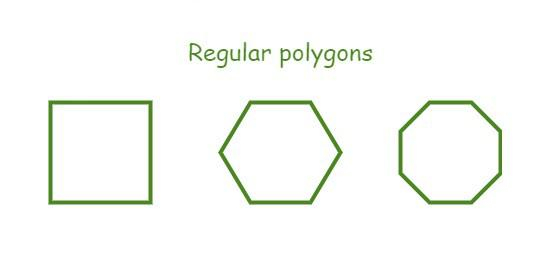### Irregular polygon

A polygon is said to be a regular polygon if it has all the interior angles and the sides have different values.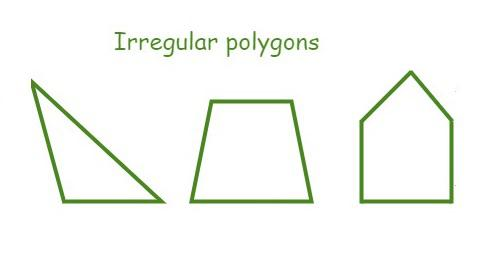### Concave polygon

A concave polygon is a polygon that has at least one interior angle greater than 180 degrees, i.e., a reflex angle.### Convex polygon

A convex polygon is a polygon that has all the interior angles of a polygon less than 180 degrees.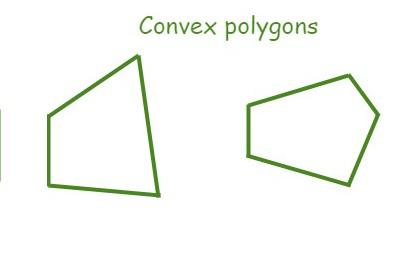### Equilateral Polygon

An equilateral polygon is a polygon whose all sides measure the same.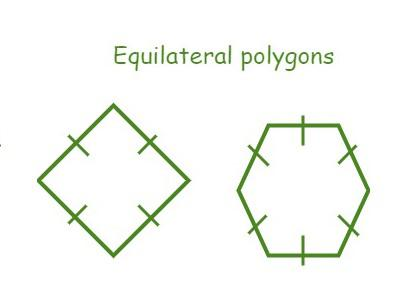### Equiangular Polygon

An equiangular polygon is a polygon whose all angles measure the same.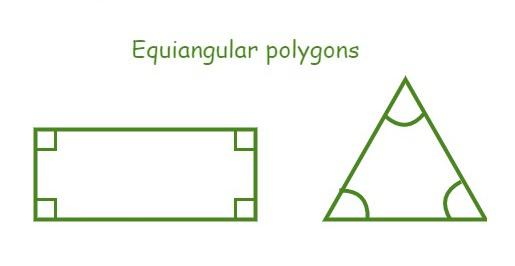## Polygon Formulae

• The sum of interior angles of a polygon is given as,

Sum of interior angles of a polygon = (n-2)×180°

where

“n” is the number of sides of a regular polygon.

• The formula for the measurement of each interior angle of a polygon is given as,

Each interior angle of a regular polygon = (n-2)×180°/n

where

“n” is the number of sides of a regular polygon.

• The measure of the exterior angles of a regular n-sided polygon is,

Each exterior angle of a regular polygon = 360°/n

where

“n” is the number of sides of a regular polygon.

• The formula for the perimeter of an n-sided regular polygon is given as,

Perimeter = n × s

where

“n” is the number of sides of a regular polygon and

“s” is the length of each side.

• The formula for the area of an n-sided regular polygon is given as,

Area of a regular polygon = (number of sides × length of one side × apothem)/2.

Area = (Perimeter × apothem)/2

Area = l/2tan(180°/n)

where

“n” is the number of sides of a regular polygon and

“l” is the apothem length.

## Solved Example based on Polygon Formula

Example 1: Calculate the perimeter and value of one interior angle of a regular heptagon whose side length is 6 cm.

Solution:

The polygon is an heptagon. So, number of sides (n) = 7

The length of each side (s) = 6 cm

We know that,

The perimeter of the heptagon (P) = n × s

P = 7 × 6

= 42 cm

Now, find each interior angle by using the polygon formula,

Interior Angle = [(n-2)180°]/n

= [(7 – 2)180°]/7

= (5 × 180°)/7

= 128.57°

Therefore, the perimeter of the given heptagon is 42 cm and the value of each internal angle is 128.57°.

Example 2: Calculate the measure of one interior angle and the number of diagonals of a regular decagon.

Solution:

The polygon is a decagon. So, number of sides (n) = 10

Now, to find each interior angle by using the polygon formula,

Interior Angle = [(n-2)180°]/n

= [(10 – 2)180°]/10

= (8 × 180°)/10

= 144‬°

We know that,

Number of diagonals in a n-sided polygon = n(n-3)/2

= 10(10 – 3)/2

= 10(7)/2 = 35.

Therefore, the value of each internal angle of a regular decagon is 144° the number of diagonals is 35.

Example 3: Calculate the sum of interior angles of a hexagon using the polygon formula.

Solution:

The polygon is a hexagon. So, number of sides (n) = 6

We know that,

The sum of interior angles of a polygon = (n-2)×180°

= (6-2)×180°

= 4×180° = 720°.

Hence, the sum of interior angles of a hexagon is 720°.

Example 4: Calculate the measures of one exterior angle and the perimeter of a regular pentagon whose side length is 9 inches.

Solution:

The polygon is a pentagon. So, number of sides (n) = 5

We know that,

The length of each side (s) = 9 inches

We know that,

The perimeter of the pentagon (P) = n × s

P = 5 × 9

= 45 inches

Each exterior angle of a regular polygon = 360°/n

=  360°/5 = 72°.

Hence, the measures of one exterior angle and the perimeter of a regular pentagon are 72° and 45 inches, respectively.

Example 5: Calculate the sum of the interior angles of a quadrilateral and an octagon.

Solution:

The given polygons are a quadrilateral and an octagon.

So, n = 4 for a quadrilateral and n = 8 for an octagon.

We know that,

Sum of interior angles of a polygon = (n-2)×180°

Sum of interior angles of a quadrilateral = (4-2)×180°

= 2 × 180° = 360°

Sum of interior angles of an octagon = (8-2)×180°

=  6 × 180° = 1080°

Example 6: Determine the area of a regular hexagon whose apothem length is 6 units.

Solution:

Given polygon is a hexagon. So, n = 6.

Apothem length (l) = 6 units

We know that,

Area = l/[2tan(180°/n)]

A = 6/[2tan(180°/6)]

= 3/tan 30°

= 3/(1/√3) = 3√3 square units.

Hence, the area of the given polygon is 3√3 square units.

## FAQs on Polygon Formulas

Question 1: What do we mean by the Polygon?

Polygon is a 2-D closed structure that is made up of three or more straight lines. A polygon consists of a minimum of three sides. Each line segment intersects with another line segment only at the vertex of Polygon. Polygon can be classified on the basis of angles. Some examples of polygons are triangles, squares, pentagons, hexagons, etc.

Question 2: Under how many categories Polygon be categorized?

On the basis of the measurement of angles and the sides of a polygon, a polygon can be classified into:

• Regular Polygon: The interior angles and the sides of the polygon are equal.
• Irregular Polygon: The interior angles and the sides of the polygon are not equal.
• Convex polygon: The interior angles of a polygon are strictly less than 180°.
• Concave Polygon: Polygons have one or more interior angles which are greater than 180°.

Question 3: Write some of the Properties of a Polygon.